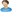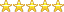Home»Computer Science» Computerization of a mathematical formula solver system

# Computerization of a mathematical formula solver system

Department: Computer Science
By:Zubair2017

Project ID: 6057
Rating:(5.0) votes: 1
Rate this project
Price:₦2000
Abstract
Geometry is one of the fundamental methods which people use to understand and explain the physical environment by measuring length, surface area and volume. For this reason, enhancing geometric thinking is very important for high-level mathematical thinking, and it should be developed with spatial interaction and manipulation in daily life (Clements& Battista, 2013 Tan, 2012). However, in traditional classrooms, geometry learning is usually conducted only through the description of text, 2D graphs and mathematical formulas on whiteboards or paper. In some important topics, such as measuring the area and volume of 2D or 3D objects, traditional teaching methods often focus too heavily on the application of mathematical formulas, and lack opportunities for students to manipulate the objects under study.  Students find it very difficult to cram all the mathematical formulas they come across in school. Therefore, the need to computerize such formulas is the aim of this project work. Since it is not easy for a human being to remember all that comes his or her way, this project work is aimed at automating some elementary mathematical formulas. The aim of this project is to develop Mathematics problem solver software that will help students to solve mathematics geometry problems using Visual Basic.net....
Preview +
Other Computer Science project topics and materials you might be interested in